# ISEE Upper Level Math : How to find the height of an eqilateral triangle

## Example Questions

### Example Question #71 : Triangles

The length of one side of an equilateral triangle is 4 centimeters. Give the height (altitude) of the triangle.

Possible Answers: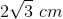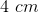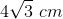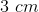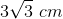Correct answer:Explanation: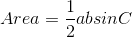,

whereandare the lengths of two sides andis the corresponding angle.

In an equilateral triangle, all of the sides have the same length, and all three angles are.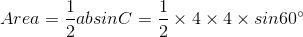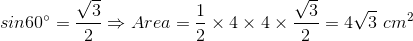Now plug this area into the alternate formula for the area of the triangle and solve for the height: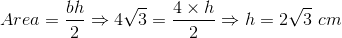### Example Question #72 : Triangles

The height of the equilateral triangle below is 5 centimeters. Give the perimeter of the triangle.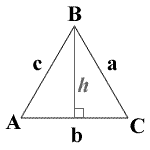Possible Answers: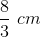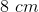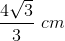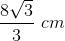Correct answer:Explanation:

In an equilateral triangle all of the sides have the same length, so all we need to do is find the length of one side.

We know that, in an equilateral triangle, all three angles measure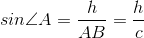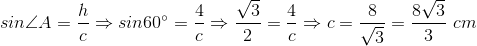### All ISEE Upper Level Math Resources